# A New Approach to ICSE Physics Part 2 Class 10 Solutions Force

## A New Approach to ICSE Physics Part 2 Class 10 Solutions Force

These Solutions are part of A New Approach to ICSE Physics Part 2 Class 10 Solutions. Here we have given A New Approach to ICSE Physics Part 2 Class 10 Solutions Force

Exercise – 1

Question 1.
(a) Define the following :

1. Rigid body
2. point of action of force
3. line of action of force
4. principle of transmissibility of force.

(b) Name four bodies which can be called rigid bodies for practical purposes.
(a)
(i) Rigid Body : “A body which does not get deformed under the action of a force or a number of forces is called rigid body,
(ii) Point Of Action Of Force : “The point on the rigid body where a force acts is called the point of action of force.”(iii) Line Of Action Of Force : “An imaginary line passing through the point of action of force and drawn in the same direction in which the force acts is called line of action of force. LM is the line of action of force.
(iv) Principle Of Transmissibility Of Force : “It states that the point of action of force can be transmitted anywhere along the line of action of the force without causing any material difference in the movement of the body.”
(b) Wood, iron rod, stone and glass are rigid bodies.

Question 2.
Under what condition (s) a body describes a motion of :

(a) translation
(b) rotation.

Conditions for

(a) Translational motion : Motion of rigid body in straight line and in the direction of applied force.
(b) Rotational motion : Rigid body should rotate about axis (or pivot).

Question 3.

(a) What do you understand by the term moment of force
(b) State two factors which determine the moment of force.

(a) Moment of force : “The turning effect of force acting on a body about an axis is called the moment of force.”
(b) Factors affecting the moment of force :

1. The magnitude of the force applied
2. The distance of line of action of the force from the axis of rotation.

Question 4.
State one way of

(a) reducing moment of force
(b) Increasing moment of force for a given force acting on a body capable of turning around a fixed point.

(a) Moment of force can be reduced by reducing the distance between force and the turning point.
(b) Moment of force can be increased by increasing the distance between force and the turning point.

Question 5.
State the law of moments.
Law of moments : In equilibrium sum of anticlockwise moments is equal to sum of clock-wise moments.
Sum of anticlock wise moments = sum of clockwise moments.

Question 6.
(a) What do you understand by the terms (i) positive moments (ii) negative moments
(b) State the absolute units of moment of force in (i) CGS system (ii) S.I. system.
(a)

1. Positive moments : If the force produces anticlock wise motion in the rigid body about turning point, it is positive moment.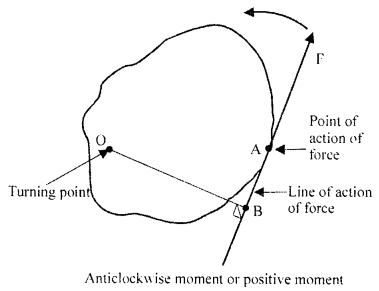2. Negative moment : If the force produces clock wise motion in the body about turning point, it is negative moment.(b) Absolute units of moment of force in

1. CGS system is dyne-cm.
2. S.I system is newton-metre (N-m)

Question 7.
(a) What do you understand by the following terms ? (i) couple, (ii) arm of couple, (iii) moment of couple
(b) State the units of the moment of couple in (i) CGS system, (ii) S.I. system.
(c) Give four examples of couple in everyday life.
(d) State the mathematical expression for the moment of a couple.
(a)

1. Couple : “Two forces acting on a rigid body are equal in magnitude opposite parallel and not along the same straight line constitute a couple.”
2. Arm of the couple : “Perpendicular distance between two forces is called arm of the couple.” i.e. AB is arm of couple.
3. Moment of the couple : “Is the product of any one of the force and the arm of couple. i.e. F × AB is moment of couple.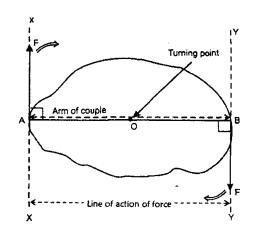(b)

1. In CGS system unit of moment of couple-dyne-cm
2. In S.I. system unit of moment of couple-N-m.

(c) Examples of couple in everyday life :

1. Steering wheel of a car.
2. Turning of a screw driver.
3. Opening and closing of water tap.
4. Winding the watch.

(d) Mathematical expression : Moment of couple = force × Arm of couple.

Question 8.
Explain the following :

(a) Jack screw is provided with a long arm.
(b) It is easier to open a door by holding it from its edge.
(c) A small boy can balance a stout man on a see-saw.
(d) The handles of a hand flour grinder (chakki) is provided near its edge.
(e) It is easier to turn a steering wheel of larger diameter than a steering wheel of smaller diameter.
(f) A wrench or a spanner has a long handle.

(a) So that there is maximum distance between force and turning point and minimum force is needed.
(b) Holding a door from edge increases the distance between force and turning point (hinge) and hence minimum force is needed.
(c) By making the smaller boy sit at longer distance from axis of rotation. Hence turning effect of force increases.
(d) To increase the distance between force and turning point and hence to apply minimum force.
(e) In this way we increase the ⊥ distance between force and point of rotation and minimum force is needed to rotate the steering.
(f) Moment of force increases by increasing the length of handle and minimum force is required.

Question 9.
The diagram along side shows a heavy roller, with its axle at O which is to be pulled on to the pavement XY by applying the minimum possible force. Draw the diagram and mark on it the point and direction in which the force should be applied?Force should be applied at A near the rim to increase the moment of force by increasing perpendicular distance OA (between force and point of rotation).Question 10.
(a) What do you understand by the term equilibrium of a body?
(b) State the condition when the body is in (i) dynamic equilibrium (ii) static equilibrium support your answer with one example each.
(c) A body is acted upon by a number of forces acting in different directions. State two conditions for a body to be inequilibrium.
(a) Equilibrium : “When two or more forces act on a rigid body such that the state of body (rest or of uniform motion) does not change, the body is said to be in equilibrium”.
(b) Condition when the body is in
(i) Dynamic equilibrium …. the body should remain in its state of uniform motion when it is under the action of forces.
Example : A train running with a constant speed is in dynamic equilibrium, because the force generated by its engine is equal to the force of friction due to rails and force of friction due to air.
(ii) Static equilibrium : The body should remain in state of rest when it is under the action of forces.
Example :
When a body is at rest on the ground the various forces are :
W = R and F = F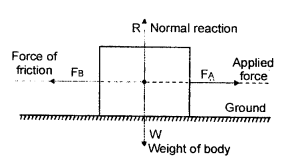(c) When a body is acted upon by a number of forces the body is to be in equilibrium.
The two conditions are :
(i) The resultant of all the translational forces should be zero.
(ii) All the clockwise moments should be equal to all the anticlock wise moments.

Question 11.
(a) What do you understand by the term centre of gravity?
(b) State the position of CG in case of the following regular bodies.

1. A triangular lamina
2. a rectangular lamina
3. a circular lamina
4. a cylinder
5. a sphere
6. a square lamina.

(a) Centre of gravity : “Is a point with in a body where the whole weight of body is supposed to act.”
(b) Position of C.G.

1. A traingular lamina is the point of intersection of medians.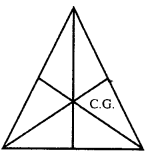2. A rectangular lamina: is the point of intersection of its diagonals3. A circular lamina is its geometric centre O4. A cylinder is a point at the centre of its axis.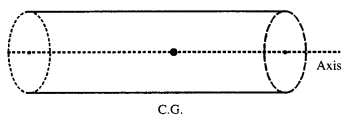5. A sphere is its geometric centre.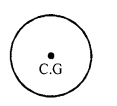6. A square is the point of intersection of its diagonals.Question 12.
(a) Is it possible to have a body whose centre of gravity is outside the body ? If so explain ?
(b) How will you determine the centre of gravity of an irregular piece of a cardboard ?
(a) Yes, the body with L shape or a Boomrang has its centre of gravity out side the body.Medians AB, CD and EF meet at point O out side the body is C.G.
(b) To find C.G of irregular body with the help of plumb line. Make holes at A, B, C, D, E sharp corners and suspand the cardboad at each hole in turn with the help of thread of plumb line draw the line below the thread.
The centre of gravity is point where maximum number of lines meet.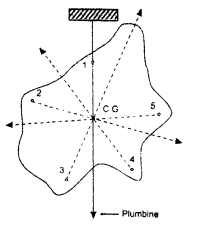Question 13.
A flat triangular cardboard equilateral in shape is suspended by passing a common pin through a narrow hole at its one corner. Draw a diagram to show its position in the state of rest. In the diagram mark the position of suspension by the letter A and centre of mass (cenre of gravity) by the letter B.
Point of suspension A and CG (B) is shown. B is the point where medians of ∆AX, DZ and CY intersect.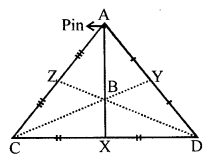Question 14.
A stone of mass ‘m’ is rotated in circular path with uniform speed by tying a strong string with the help of your hand. Answer the following questions.

(a) Is the stone moving with a uniform or variable speed ?
(b) Is the stone moving with a uniform acceleration ? What is the effect of acceleration ? In which direction does the acceleration act ?
(c) What kind of force acts on the stone and state its direction ?
(d) What kind offorce acts on the hand and state its direction?

(a) Uniform speed.
(b) Yes, the stone is moving with uniform acceleration.
Effect of accelerated motion is it makes the body move in circular path. Acceleration acts at right angle to the radius of circle.
(c) Centripetal force acts on stone. Its direction is towards the centre of circle.
(d) Centrifugal force acts on hand. Its direction is opposite to the direction of centripetal force i.e. away from centre of circle along the radius.

Question 15.
State whether the following statements are true or false :

(a) on deformation of a body,Jhe position of its centre of gravity does not change.
(b) The centre of gravity of freely suspended body is always vertically below the point of suspension.

(a) False
(b) True

Question 16.
Define or explain

(a) circular motion
(b) centripetal force
(c) centrifugal force

(a) Circular Motion : “Motion of a body around a circular path with uniform speed, but variable velocity, such that it is acted upon by a uniform acceleration is called circular motion.”
(b) Centripetal Force : “A force which is directed towards the centre of a circular path and always acts at right angles to the direction of motion, along the circular path is called centripetal force.”
(c) Centrifugal Force : “The force acting on a body away from centre of its circular path is called Centrifugal Force.”

Question 17.
Give an example of a body moving with a uniform speed, but has an accelerated motion.
Motion of moon around the earth is example of moon (with uniform speed and accelerated motion). Gravitational force of the earth provides necessary centripetal force.

Question 18.
Compare uniform circular motion and uniform linear motion.
Uniform Circular motion :

1. Speed of body is uniform.
2. It is accelerated motion.
3. Velocity of body is variable.
4. External force is needed.

Uniform Linear motion :

1. Speed is uniform.
2. Acceleration is zero.
3. Velocity is constant.
4. No external force is needed.

Question 19.
Explain the motion of moon around the earth.
The moon moves around the earth with uniform speed in a circular path. The centripetal force for its motion is provided by earth.

Question 20.
With reference to the magnitude of force and its direction, how does centripetal force differ from centrifugal force.
Both centripetal force and centrifugal force have same magnitude but they are opposite in direction.

Multiple Choice Questions

Tick ( ✓ ) the most appropriate option

1. The point of action of force on a rigid body is :
(a) Fixed point on rigid body
(b) Fixed point but can be transferred any where along the line of action of force.
(c) Fixed point but can be transferred anywhere along the direction of force.
(d) Fixed point, but can be transferred anywhere opposite to the direction of force.
(b) Fixed point but can be transferred any where along the line of action of force.

2. The turning effect produced in a rigid body around a fixed point by the application of force is called;
(a) turning force
(b) movement of force
(c) moment of couple
(d) none of these
(b) movement of force

3. The unit of moment of force in SI system is :
(a) Nm
(b) dynecm
(c) dynem
(d) Ncm
(a) Nm

4. The moment of couple is mathematically the :
(a) product of one force and the perpendicular distance between two forces
(b) product of both forces and the perpendicular distance between them
(c) product of one force and tKe perpendicular distance between the point of application of force and turning point.
(d) None of the above.
(a) product of one force and the perpendicular distance between two forces

5. The condition for equilibrium is:
(a) the resultant of all the forces acting on the body be zero only.
(b) the resultant of moments of all the forces acting on the body about the turning point should be zero.
(c) both (a) and (b)
(d) none of the above
(b) the resultant of moments of all the forces acting on the body about the turning point should be zero.

6. A body is acted upon by two unequal and opposite forces along different lines of action of force. The body will have
(a) only rotatory motion
(b) only translatory motion
(c) both (a) and (b)
(d) neither (a) nor (b)
(a) only rotatory motion

7. A force F acts on a rigid body capable of turning around a fixed point. The moment of force depends upon
(a) magnitude of force F
(b) magnitude of perpendicular distance between the point of action of force and the turning point
(c) both (a) and (b)
(d) none of these
(c) both (a) and (b)

8. The centre of gravity of a cricket ball is at:
(a) its geometric centre
(b) at its bottom touching the ground
(c) its top most point
(d) at any point on its surface
(a) its geometric centre

9. A body is describing a uniform cicular motion. Which of the following quantities is/are constant
(a) speed
(b) acceleration
(c) velocity
(d) both (a) and (b)
(a) speed

10. In a uniform circular motion :
(a) speed of body continuously changes because the direction of motion changes
(b) velocity of body continuously changes because the direction of motion changes
(c) the motion of body is accelerated
(d) both (b) and (c)
(d) both (b) and (c)

Numerical Problems on Moment of Force

Practice Problems 1

Question 1.
A force of 50 dynes acts on a rigid body, such that the perpendicular distance between the fulcrum and the point of application of force is 75 cm. Calculate the moment of force.
Force F = 50 dynes
⊥ distance = 75 cm
∴ Moment of force = F × ⊥ distance
= 50 × 75 = 3750 dynecm

Question 2.
The perpendicular distance between the point of application of force and the turning point is 1.75 m, when a force of 80 N acts on a rigid body. Calculate the moment of force.
⊥ distance = 1.75 m
Force F = 80 N
∴ Moment of force = F × ⊥ distance
= 80 × 1.75 = 140.00
= 140 Nm

Practice Problems 2

Question 1.
A force of 50 N produces a moment of force of 10 Nm in a rigid body. Calculate the perpendicular distance between the point of application of force and the turning point is 45 cm.
F = 50 N
Moment of force =10 Nm
⊥ distance = ?
Moment of force = F × ⊥ dist.
10 = 50 × ⊥ distance.
∴ ⊥ distance 10/50 = 0.2 m

Question 2.
Calculate the force which will produce a moment offorce of 1575 dynecm, when the perpendicular distance between point of application of force and turning point is 45 cm.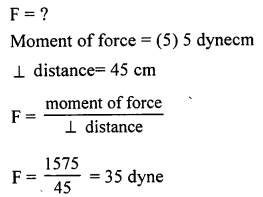Practice Problems 3

Question 1.
A couple of 15 N force acts on a rigid body, such that the arm of couple is 85 cm. Calculate the moment of couple in S.I. system.Question 2.
Calculate the length of the arm of couple, if a force of 13 N produces a moment of couple of 14.3 Nm.Question 3.
Two forces each of magnitude 2N act vertically upward and downward respectively on two ends of a uniform rod of length lm, freely pivoted at its centre. Determine the resultant moment of forces about the mid-point of the rod.
As the two equal forces 2N are acting at the ends of pivoted rod AB. These constitute a couple in anti-clockwise direction.Practice Problems 4

Question 1.
The diagram along side shows a force F = 5 N acting at point A produces a moment of force of 6 Nm about point O. What is the diameter of the wheel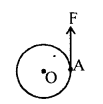Question 2.
The diagram alongside shows a force F acting at point A, such that it produces a moment of force of 20 Nm in clockwise direction. Calculate the magnitude of force F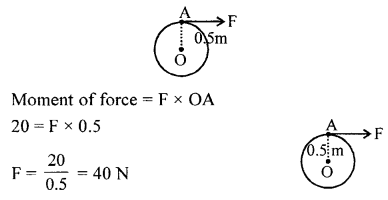Practice Problems 5

Question 1.
Study the diagram alongside and calculate the moment of couple.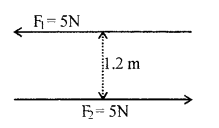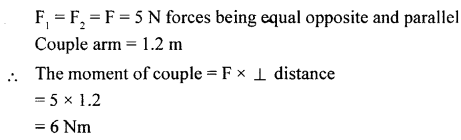Question 2.
Two forces F1 and F2 are applied on a circular body such that moment of couple is 9 Nm in CWD. Calculate the radius of circular body.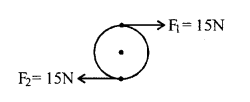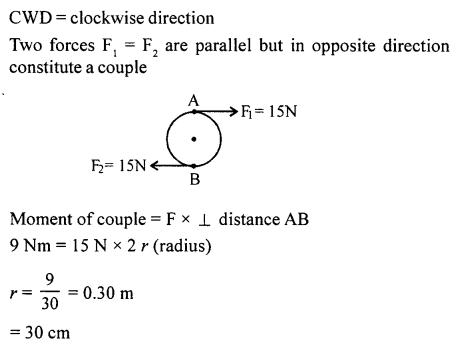Question 3.
Two forces F1 = F2 are applied on a wheel of 1.5 m radius, such that moment of couple is 30 Nm. Calculate the magnitude of each of the force.Anticlockwise directionF1 = F2 = F in opp. direction and are parallel
Hence constitute a couple
moment of couple = F × diameter AB
30 = F
30 = F × (2 × 1.5)
F= 30/3 = IO N

Practice Problems 6

Question 1.
A uniform metre scale is balanced at 60 cm mark, when weights of 5 gf and 40 gf are suspended at 10 cm mark and 80 cm mark respectively. Calculate the weight of the metre scale.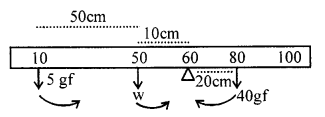Let w be the mass of metre scale acting a mid point 50 cm
Clock wise moment = 40 × (80 – 60)
= 40 × 20 = 800 g f cm …….(i)
Anticlockwise moments
= 5 × (60 – 10) + w × (60 – 50)
(250 + 10 w) gf cm ……..(ii)
Anticlockwise moment = clock wise moment
250+ 10 w = 800
10 w= 800 -250 = 550
w = 550/10 = 55 gf

Question 2.
A uniform metre scale is balanced at 20 cm mark, when a weight of 100 gf is suspended from one end.Where must the weight be suspended ? Calculate the weight of the metre scale.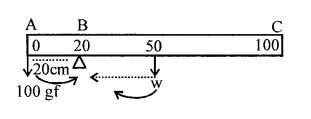Let w be the weight of metre scale.As the scale is balanced at 20 cm and 100 gf is suspended on one end (0 mark). The weight of longer arm i.e. BC is balanced by 100 gf
∴ CW moment = A.C.W moment
W × 30 = 20 × 100
W = 2000/30 = 66.66 gf
wt. of 100 gf should be suspended at zero mark.

Question 3.
A uniform metre scale balances horizontally on a knife edge placed at 55 cm mark, when a mass of 25 g is supported from one end. Draw the diagram of the arrangement. Calculate mass of the scale.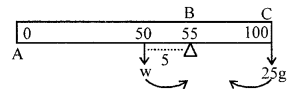As the metre scale is balanced at 55 cm mark i.e. large arm is balanced by arm BC and 25 g is suspended at one end i.e. at 100 cm mark
∴ Anticlockwise moment = clockwise moment
W × (55 – 50) = 25 × (100 – 55)
W = 25 × 45 / 5 = 225g

Practice Problems 7

Question 1.
A uniform metre scale of weight 50 gf is balanced at the 40 cm mark, when a weight of 100 gf is suspended at the 5 cm mark. Where must a weight of 80 gf be suspended to balance the metre scale ?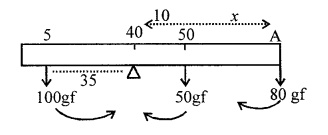Let a wt. of 80 gf be placed at a distance x from 40 cm mark.
Clock wise moments = Anticlock wise moment
(80 × x) + 50 × (50 – 40) = 100 × (40 – 5)
80 x +500 = 3500
80 x = 3500 – 500 = 3000
x = 3000/80 = 37.5 cm
∴ A is at 40 + 37.5 = 77.5 cm mark

Question 2.
A see-saw 8 m long is balanced in the middle. Two children of mass 30 kgf and 40 kgf are sitting on the same side of the fulcrum at a distance of 1.5 m and 3.5 from the fulcrum respectively. Where must a lady weighing 60 kgf sit from the fulcrum, so as to balance the see-saw ?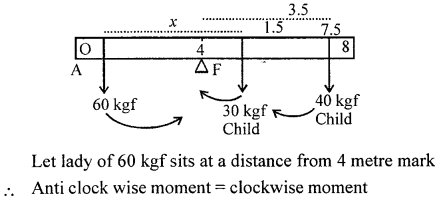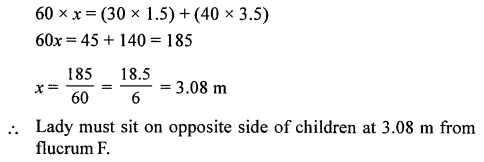Question 3.
A uniform wooden beam AB, 80 cm long and weighing 250 gf, is supported on a wedge as shown in the figure. Calculate the greatest weight which can be placed on end A without causing the beam to tilt.Question 4.
Figure shows a uniform metre rule weighing 100 gf, pivoted at its centre ‘O’. Two weights of 150 gf and 250 gf hang from the metre rule as shown. Calculate :

(a) Total C.W. moment about ‘O’.
(c) Differentiate of C.W. andA.C.W. moments.
(d) The distance from ‘O’ where a 100 gf weight should be suspended to balance the metre scale.Question From ICSE Examination Papers

2011

Question 1.
(a) Where does the position of centre of gravity lie for

1. a circular lamina ?
2. a triangular lamina ?

1. Its centre of Gravity lies at its Geometrical Centre
2. The point of intersection of diagonals.

(b) A uniform metre scale canbe balanced at 70.0 cm mark, when a mass of 0.05 kg is hung from 94.0 cm mark.

1. Draw the diagram of the arrangement.
2. Find the mass of metre scale.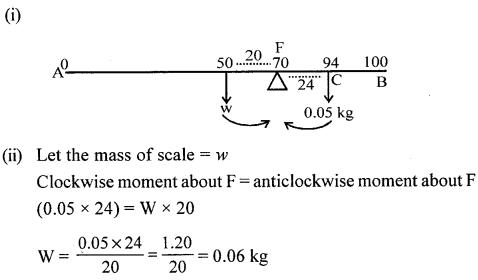2012

Question 2.
(a) A boy of mass 30 kg is sitting at a distance of 2 m from the middle of a see-saw. Where should a boy of mass 40 kg sit so as to balance the see-saw ?
(i) What is meant by the term ‘moment of force’ ?
(ii) If the moment of force is assigned a negative sign, then will the turning tendency of the force be clockwise or anitclockwise?
(c) (i) Which of the following remain constant in uniform circular motion : speed or velocity or both ?
(ii) Name the force required for uniform circular motion. State the direction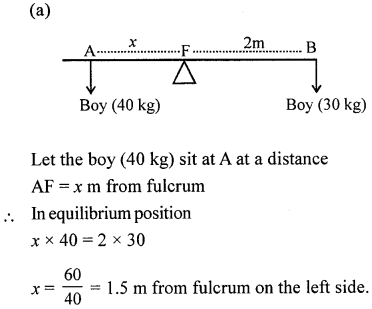(b)

1. Moment of force : “The turning effect of force acting on a body about on axis is called moment of force.”
2. Clockwise is taken negative.

(c)

1. Speed-remains constant.
2. Centripetal force. It acts towards the centre of circular path at right angles to the direction of motion.

2013

Question 3.
(a)
(i) Where is the centre of gravity of a uniform ring situated.
(ii) ‘The position of centre of gravity of a body remains unchanged even when the body is deformed. ’State whether the statement is true or false.
(b) With reference to their direction, how does centripetal force differ from centrifugal force ?
(a)
(i) At the centre of ring, (where there is no mass)
(ii) False.
(b) Both force have equal magnitude but in oppose direction.
i.e. contripetal force towards the centre of circular path but centrifugal force acts away from centre.

2014

Question 4.
(a) Is it possible to have an accelerated motion with a constant speed ? Explain.
Yes, a body moving in circular path has accelerated motion with constant speed.
(b) Two forces each of 5 N act vertically upwards and downwards respectively on the two ends of a uniform metre rule which placed at its mid point as shown in the diagram. Determine the resultant moment of these forces about the mid-point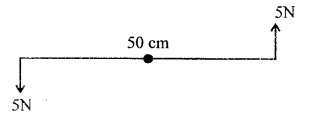2015

Question 5.
(a) On whatfactor does the position of the centre of gravity of a body depend ?
(b) Name the factors affecting the turning effect of a body :
(c) (i) Define equilibrium.
(ii) In a beam balanced when the beam is balanced in a horizontal position, it is in _____ equilibrium.
(d) Explain the motion of a planet around the Sun in a circular path.
(a) The whole weight of body should act at that point.
(b) Factors affecting the turning effect :

1. force applied
2. ⊥ distance between turning point and point of application of force.

(c)

1. Equilibrium : “When two or more forces act on a rigid body such that the state of body (rest or of uniform motion) does not change, the body is said to be in equilibrium.
2. Static

(d) A planet moves around the Sun in a circular path and the gravitational force of Sun acts on planet and provides necessary centripetal force.

2016

Question 6.
(a) A stone of mass ‘m’ is rotated in a circular path with a uniform speed by tying a strong string with the help of your hand. Answer the following questions :

1. Is the stone moving with a uniform or variable speed ?
2. Is the stone moving with a uniform acceleration ? In which direction does the acceleration act?
3. What kind of force acts on the hand and state its direction?

1. The stone is moving with uniform speed as given in the question.
2. Uniform acceleration. It acts at right angles to the direction of motion of stone.
3. The force which acts on the hand is the Centripetal force. It is directed towards the hand along the string.

Question 1.
Define force.
Force : “Is an external agent which changes or tends to change the state of rest or of uniform motion of a body in straight line or bends or deforms a body.”
Or
Force : “Is the which produces or tries to produce a change in the state of rest or of uniform motion or shape of the body.”

Question 2.
What are contact forces ? Give four examples of contact forces.
Contact forces : “The forces which act on another body through some connector are called contact forces.”
Or
“The forces which act on bodies when they are in actual contact.”
Examples :

1. Force of friction
2. Force of tension.
3. A player can set a ball in motion by hitting it with his foot or by pushing it with a bat.
4. Pulling a rubber bond to increase its length.

Question 3.
Among the forces of pull or push which can be applied only through (a) a rigid connect or (b) non-rigid connector. Give an example in support of your answer.
(a) Pull and push both can be applied through a rigid connector.
e.g. we can pull a table with a rigid rod. We can also a table with a push rigid rol (connector).
(b) Non-rigid connector can not be applied to push a table i.e. a rope cannot push a table to move it from one place to other. Only pull can be applied through non-rigid connector.

Question 4.
What are forces at a distance ? Give three examples
Forces at a distance : “A force which does not make a direct contact with a body, but acts through space is called force at a distance or A Non-Contact Force.”
Three examples :

1. Gravitational force acting on a falling body.
2. Electrostatic force between two charged particles.

Question 5.
Classify the following as contact and non-contact forces :

1. A horse pulling a cart
2. A magnet attracting a piece of iron.
3. A freely falling stone.
4. A boy shooting an arrow from a bow.
5. Tides in the sea.
6. A speeding car stops when an engine is switched off.
7. Two negatively charged ball repelling each other.
8. Crushing paper into paper ball.

1. A horse pulling a cart → Contact force.
2. A magnet attracting a piece of iron → Non contact force.
3. A freely falling body → Non-contact force.
4. A boy shooting an arrow from a bow → Contact force.
5. Tides in the sea → Non-contact force.
6. A speeding car stops when an engine is switched off → Contact force.
7. Two negatively charged ball repelling each other → Non-contact force.
8. Crushing paper into paper ball → Contact force.

Question 6.
A brass ball is hanging from a stiff cotton thread. Draw a labelled diagram showing the forces acting on the brass ball and the cotton thread.
Forces acting on the brass ball

1. Weight w acting vertically downward.
2. Tension T in the thread in upward direction.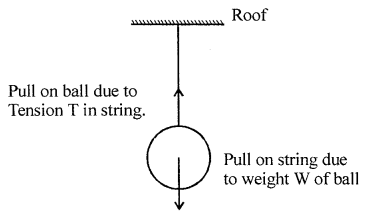Question 7.
A spring is attached to a hook in a wall and is pulled outward. Draw a labelled diagram showing the forces acting on the spring.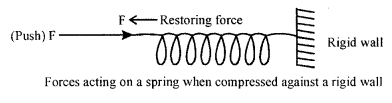When spring is pulled outward restoring force acts towards the wall.

Question 8.
State three characteristics of non-contact forces.
Characteristics of non-contact forces :

1. Non – contact forces can pass through any kind of medium. They can pass through vacuum.
2. Gravitational force is always attractive in nature.
3. Magnitude of non-contact forces on two bodies is inversely proportional to the square of distance between their centres.

Question 9.
The distance between two bodies is doubled. How is the magnitude of gravitational force between them affected?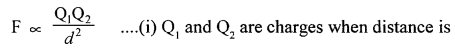Question 10.
(a) What do you understand by “balanced forces” ?
(b) Draw a diagram for balanced forces. When a force is applied on a wooden block, such that it does not move.
(c) Name two pairs of forces which are numerically equal to each other in question.
(a) Balanced force : “When a number of forces act on a body simultaneously such that their resultant is zero and hence, the body continues in its state of rest or of uniform motion in a straight line, then the set of forces acting on the body are said to constitute balanced forces.”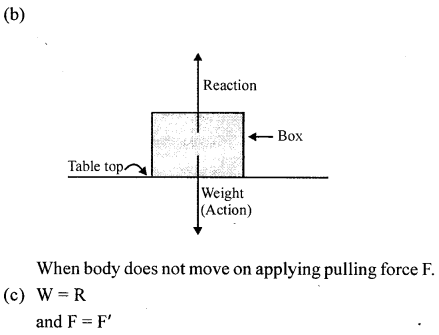Question 11.
(a) What do you understand by the term “unbalanced forces”.
(b) Draw a diagram showing various forces acting on a box which is pushed, such that it starts sliding.
(c) In question 11 (b) which pair of forces is (i) balanced (ii) unbalanced ?
(a) Unbalanced forces : “The set of forces acting on a body simultaneously, such that their resultant force is not zero and the body does not continue in the state of rest or of uniform motion in a straight line, the forces are called unbalanced forces.”
(b) fig.
(c)

1. Balanced forces acting on box are R = W normal reaction equal to weight of box.
2. Unbalanced forces are F’ < F i.e. pushing force (F) is greater than force of friction F’ and hence the box moves to the right in the direction of force.

Question 12.
State three effects which an unbalanced force can produce.
Effects produced by unbalanced force :

1. It produces motion in the body.
2. It can stop a body in motion or it slows down it.
3. It changes the direction of motion of body.

Question 13.
Name the type of force (balanced or unbalanced) which changes the shape of a rubber ball on compressing.
It is unbalanced force
Compressing force F > F ‘
F ‘ is restoring force.Question 14.
Name the unbalanced force which

1. allows a body to fall down from a height
2. stops a moving car, when its engine is shut off.

1. Down ward force (mg) = weight of body is more that upthrust (upward force or resistance due to air).
2. Force of friction due to ground and air on car is greater than the moving force acting on car.

Question 15.

1. Name and define absolute units of force in C.GS. and S.I systems.
2. Derive a relation between newton and dyne.Question 16.

1. Name and define gravitational units of force in CGS and S.I. system.
2. A force of 5 kgf acts on a body. Express the force in (a) gf (b) newtons (c) dynes [Take g = 10 ms-2]

Gravitational unit of force in CGS system is gram force.
Gram force (gf) : “Is force which produces an acceleration due to gravity (980 cms-2) in a body of mass 1 gram”.Multiple Choice Questions

1. Which of the following is not contact force ?
(a) Frictional force
(b) Normal reaction
(c) Electrostatic force
(d) Force of compression in a spring.
(c) Electrostatic force

2. Which of the following is not non-contact force
(a) Gravitational force
(b) Force of tension
(c) Electrostatic force
(d) Magnetic force
(b) Force of tension

3. The mathematical expression for force is(d) all of these

4. 1 kgf is equal to :
(a) 1 kg × 9.8 ms-2
(b) 1 kg × 1ms-2
(c) 1 kg × 980 cms
(d) 1 kg × 98 ms-2
(a) 1 kg × 9.8 ms-2

5. A wooden block is placed on a table top and then gently pulled such that it does not move. In this situation
(a) applied force on the block is equal to frictional force only.
(b) applied force on the block is equal to the weight of block only.
(c) weight of block is equal to the normal reaction only.
(d) both (a) and (c)
(d) both (a) and (c)

6. A cricket ball rolling down the ground stops after travelling some distance. The balanced force acting on the ball is
(a) gravitational force
(b) frictional force of ground
(c) frictional force of air
(d) both (b) and (c)
(d) both (b) and (c)

7. With the increases in distance between the centres of two masses, the gravitational force between them :
(a) increases
(b) decreases
(c) remains same
(d) depends upon the magnitude of mass.
(b) decreases

8. A stone is projected vertically upward. As the stone rises up the gravitational force acting on it
(a) increases
(b) decreases
(c) remains same
(d) none of these
(b) decreases

Numerical Problems

Question 1.
How much acceleration will be produced in a body of mass 10 kg acted upon by a force of 2 kgf ? [g = 9.8 ms-2]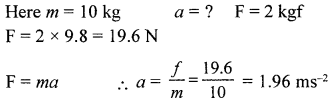Question 2.
The weight of a body on earth is 98 N where acceleration due to gravity is 9.8 ms-2. What will be its (i) mass and (ii) weight on the moon where acceleration due to gravity is 1.6 ms-2 ?Question 3.
What force will produce on acceleration of 3.5 m/s2, in a body of mass 12.5 kg ?
F = m × a
= 12.5 × 3.5 = 43.75 N

Question 4.
Calculate the mass of a body, when a force of 525 N, produces on acceleration of 3.5 m/s2.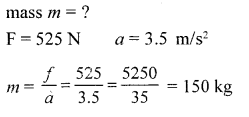Question 5.
The engine of a truck of mass 4t, produces a force of 7500 N. Calculate the acceleration of the truck produced by the engine.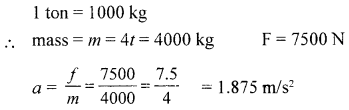Question 6.
A cyclist and a person on a moped are acted upon by the same force, If the acceleration produced by the cyclist is only 1/25 th that of the moped, calculate the ratio of the masses of the cyclist and the moped.Question 7.
Two blocks of different metals, but identical in shape and size, are acted upon by equal forces, such that the acceleration of the second block is 3 times more than that of the first block. What is the ratio of the mass of the second block with respect to that of the first block ?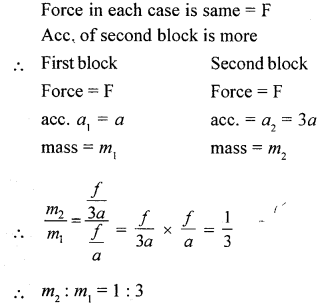Question 8.
A force of 100 N gives a body of mass m}, an acceleration of 5 ms/s2. The same force can give an acceleration of 40 m/s2. The same force can give acceleration of 40 m/s2 to another body of mass m2 If both the bodies are tied together and acted upon by the same force as above what acceleration is produced in the combination ?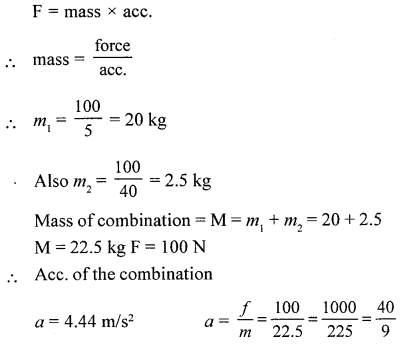Question 9.
A force of 500 N acts on a body of mass x and gives it an acceleration of 200 m/s2. The same force acting on another body of mass y gives it an acceleration of 40 m/ s2. If x and y are tied together and the same force as above acts on them, what acceleration is produced in the combination ?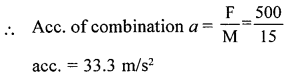Question 10.
A vehicle is acted upon by a force of 120 kgf. If the mass of vehicle is 80 kg. What is the acceleration of the vehicle? [take g = 10 ms-2]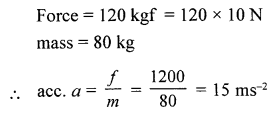Question 11.
A force of 64 kgf produces an acceleration of 2 ms-2 in a body. What is the mass of the body ? (Take g = 10 ms-2)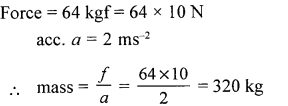Question 12.
A hockey ball of mass 100 g is at rest. It is hit by a player, such that it leaves the hockey stick with a velocity of 40 ms-1. If the time of contact of hockey with the ball is 0.01s. Calculate

1. the acceleration of the ball
2. force acting on the ball.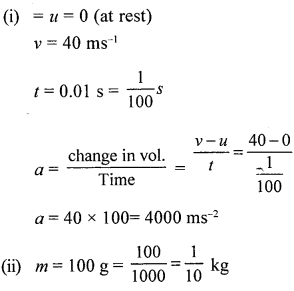Question 13.
A car of mass 600 kg running at a constant speed of 30 ms-1 is brought to rest in 3 secs. Calculate

1. acceleration of car
2. force acting on the car.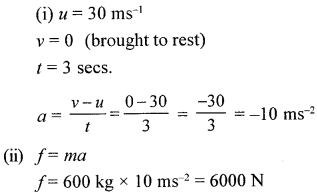Question 14.
A stone is dropped from a height of 80 m on the surface of moon takes 10s. to reach to the ground level. If the mass of stone is 100 kg. Calculate

1. acceleration due to gravity of moon
2. force acting on the stone.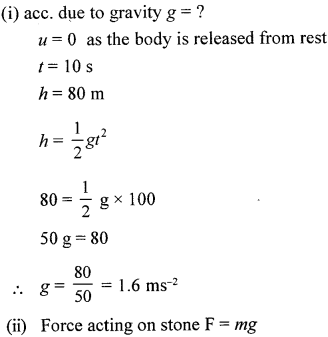Question 15.
A car starting from rest covers a distance of 200 m in 5 s with a uniform acc. If mass of car is 800 kg calculate

1. Acceleration acting on car
2. force developed by the engine of the car.Question 16.
A bullet of mass 50 g strikes a wooden log with a velocity of 200 ms-1 and is brought to rest in 0.1 s. Calculate

1. retardation
2. distance penetrated by bullet in the wood
3. retarding force.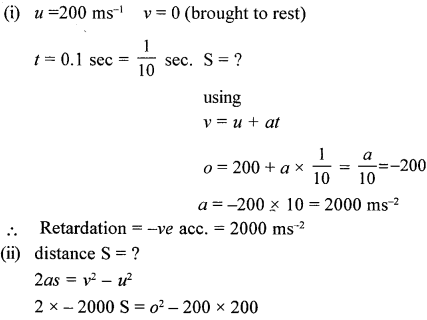Question 17.
A train of mass 200 t running at a constant speed of 20 ms-1 is brought to rest in 1 min and 40 s. Calculate :

1. retardation
2. distance covered by the train before stopping
3. retarding force.